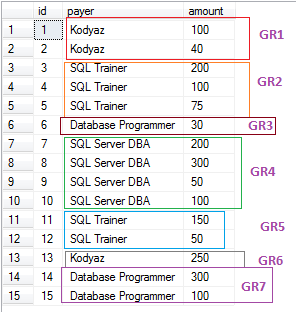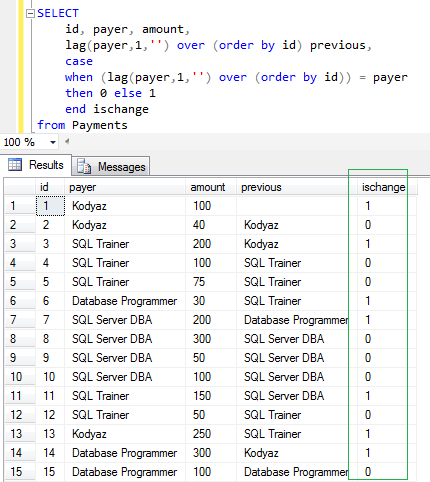﻿ SQL Server Lag Function to Group Table Rows on Column Value ChangesSQL Server and T-SQL Development Tutorials Development resources, articles, tutorials, code samples, tools and downloads for ASP.Net, SQL Server, Reporting Services, T-SQL, Windows, AWS, SAP HANA and ABAP

# SQL Server Lag Function to Group Table Rows on Column Value Changes

In this SQL Server tutorial, database developers will use SQL Lag() function to group subsequent table rows on changes of a specific column value. And then database programmers will use SQL Server aggregate functions like max(), min(), sum() and count() with "partition by" clause to find count or rows, minimum or maximum value of a row data, or sum of a column of each group.

This SQL tutorial will help developers to find maximum or minimum values of group of row columns that are sharing the same value on a specific column. I know it is difficult to explain like this, it will be more clear for SQL programmers when I illustrate the case with sample data. But in short, I group rows following one an other according to a column value. If the column has the same value for two subsequent rows, then they are in the same group or category.

First of all, in this Transact-SQL tutorial, I'll share how SQL developers can group table rows into groups according to their values compared with subsequent rows. For grouping I need to read the previous or next rows values on the criteria field.
For this task to read previous row's value in the sequence, I will use SQL Lag() function which is introduced to SQL programming for developers with SQL Server 2012.
Another similar SQL function is SQL Lead() function.

Here is my sample SQL table data for this tutorial. SQL developers can find the table DDL script and DML script for sample data insert

If you query table data, you will see the ordered by id as follows.
I marked the groups with colored rectangles to distinguish each group from other.
As you can guess, I group rows according to "payer" column value.If the next payer is different than the current row payer, then the current group is enclosed and a new group is starting.

Although a value is repeated later so that the sequence is distrupted or divided by different payer values, this means those repeating values belongs to a different group. On the screenshot, Group 1 and Group 6 are sharing same payer but they form different groups. Just like the Group 3 and Group 7, and the same is valid for Group 2 and Group 5 pairs.

What is important in this SQL problem is that:
SQL table rows are in a sequence defined by "id" column,
Grouping among subsequent rows are defined by "payer" column

Let's now mark the rows where a change has occured in the sequence of rows by Id column. Note that SQL Server Lag() function is used.

Check the rows where ischange column value is equal to 1.
On these rows the payer value is different than the previous column value.
On the other hand on rows where the ischange column has the value 0, the payer and the previous columns have the same value.I used above SQL Select statement in a SQL CTE expression to make the reading easier instead of using sub-queries.

Please pay attention to the second CTE expression which is named as "tbl".
On this multiple-CTE structured Select statement, the GroupNo column shows sequentially increasing order number of changing groups.

As SQL programmers will see, our problem was to find the maximum or minimum values according to Group Number field of the below result set.After identifying groups and giving group numbers to differentiate them from other, summing or listing the min or max values is an easy task to complete.

SQL Server developers can use SQL aggregate function with Over() (Partition By ...) clause after the release of SQL Server 2005. Partition By in aggregate functions enables programmers to apply these SQL functions only on that partition as a subset of the all resultset.

And here is the output of the above SQL CTE Select statement where SQL Lag() analytic function, and aggregate functions with Partition By clause is used.
On the screenshot, you can see minimum amount, maximum amount, total amount and number of rows as transaction count on each group where the groups are defined by payer column value changes according to following row in order.I hope SQL programmers will find this SQL tutorial useful

If you do not know these new functions SQL Lag() function, SQL Lead() function, and others shipped with SQL Server 2012, please review the SQL tutorial SQL Analytic Functions new in SQL Server 2012.
For the SQL Server aggregation functions with Partition By clause, you can also review SQL tutorial at SQL Count Function with Partition By Clause

SQL Server